# The inverse of 3 x 3 matrices with matrix row operations

## The inverse of 3x3 matrices with matrix row operations

From our lesson about the 2x2 invertible matrix we learnt that an invertible matrix is any square matrix which has another matrix (called its inverse) related to it in a way that their matrix multiplication produces an identity matrix of the same order. In general, this condition of invertibility for a $n \times n$ matrix $A$ is defined as:

$A \cdot A^{-1}=A^{-1}A \cdot A =I_{n}$

Remember that, we call them nxn matrices because they are all square matrices (same numbers of rows and columns). And so, following the condition from equation 1, we have that if we define a 2x2 matrix $X$, the condition for the inverse 2x2 matrix is written as:

$X \cdot X^{-1} =X^{-1} \cdot X=I_{2}$

So let us start this lesson with a quick review on how calculate the inverse of a 2x2 matrix since it will help us understand the standard (or formal) methodology to compute the inverse of a 3x3 matrix.

#### How to find the inverse of a matrix

Recall from our lesson on the the inverse of a 2x2 matrix that given a 2x2 matrix, which we will call $X$ just as the one shown below: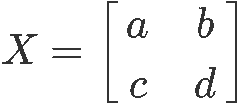We can find the inverse of a matrix such as $X$ by computing its determinant, and then dividing a modified version of the original matrix by this number in the next manner: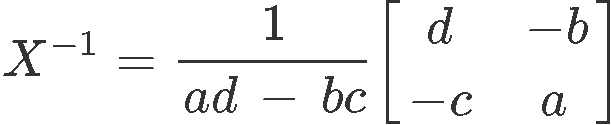Notice that the first factor in the right hand side is composed of a division with the determinant of the original 2x2 matrix in place of the denominator. This comes from our general equation for the determinant of a 2x2 matrix, which is mathematically defined as:

$det (X)=ad-bc$

The second factor in the right hand side of equation 4 is what we called before the modified version of the original matrix, where we have swapped the elements of the top left corner with the one in the bottom right corner, and then multiplied by -1 the elements left in the matrix which werent swapped (basically, the elements in the remaining two other corners).

So this is pretty much the principle behind the formal methodology to compute the inverse of any square matrix, not only those of second order (with two rows and two columns, or simple 2x2). For the case of matrix $X$ defined in equation 3, its inverse receives the name of $X^{-1}$.

This particular notation for matrix inverses is interesting to study, since the notation itself hints us that what is happening when we invert matrices is a division of matrices. Remember that this is not true, division of matrices does not exists in itself, and so, never be fooled to think that you could simply divide a matrix with another one (or even divide a number by a matrix) in order to obtain an inverse.

In conclusion, a matrix inversion comes to represent an equivalent operation to algebraic division in the sense that it allows the cancellation of matrices to take place when used as variables in solving systems of equations or even in simple matrix multiplications. But remember, this operation is NOT a division in itself, and so, is always called inversion.

What is this cancellation of matrices that we are talking about? Well, this is actually how matrix inversion comes to be an important part of linear algebra, and we have explained this already with the next example:
Imagine you have a matrix multiplication defined as $A \cdot B = C$, where all $A$, $B$, and $C$ are all square matrices of the same order (same dimensions) and $A$ and $C$ are both known. Then you are asked to find out what the $B$ matrix is.
Intuitively you will think about dividing out matrix $A$ from both sides of the equation in order to cancel it out from the left hand side and solve for $B$. This is the method that we would use if they were regular variables after all, the problem is, these are matrices and division of matrices does not exist! Therefore, in order to cancel matrix $A$ from the left hand side of the equation we obtain its inverse and multiply it to both sides as follows:

$A \cdot B = C$

$A^{-1} \cdot A \cdot B=A^{-1} \cdot C$

$I_{n} \cdot B=A^{-1} \cdot C$

$B=A^{-1} \cdot C$

Three important things can be observed here:

First, this solution allows us to appreciate the equivalency of inversion of matrices to algebraic division when solving equations where matrices are taken as variables.

Second, any matrix multiplied by an identity matrix gives a result the non-identity matrix itself.

And third, we can cancel out matrices in equations like this thanks to a special case of matrix multiplication. Remember, in general, matrix multiplication IS NOT commutative, but for square matrices that are inverses of each other, this property does not apply. Just go back and take a look at equation 1, notice how no matter in which order the factors are arranged, the matrix multiplication of two matrices which are inverse of each other produces an identity matrix $I_{n}$ of the same dimensions as the two original matrices. The same applies to the multiplication of a square matrix and the identity matrix of its same order: any matrix multiplied with an identity matrix of the same dimensions (both need to be square matrices of course) will produce the same non-identity matrix from the multiplication, no matter the order in which they were multiplied. And so, we can conclude that $B$ is equal to the inverse of $A$ times $C$.

Now, let us take this knowledge as basis in order to understand how to find the inverse of a 3x3 matrix. Although the process in itself get complicated as more elements are found inside the matrix, the principle from equation 4 remains even for matrices of greater dimensions.

#### How to find the inverse of a 3x3 matrix

Let us define a matrix named $A$ as follows: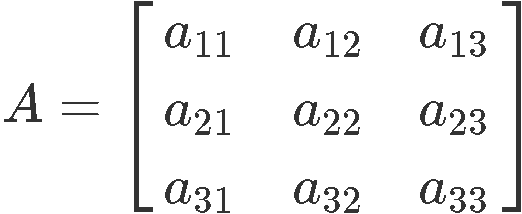In order to explain the formal method to obtain the inverse of 3x3 matrix $A$, let us see the complete equation formula for its calculation first, compare it to equation 4 and then explain step by step how it is worked out.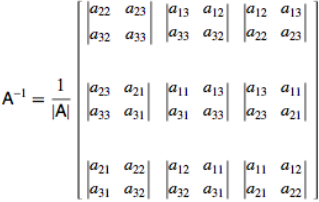For starters, in equation 8 we can see how this formula also contains a product of the division by the determinant of the original matrix and a modified version of the original matrix, just as for the case of the inverse of a 2x2 matrix.

At this point we know how to the determinant of a 3x3 matrix (with general & shortcut method), and so, the first factor on the right hand side of equation 8 should be easy to obtain.
The complicated on the calculation of a 3x3 inverse matrix comes from the second factor in the right hand side: the modified version of the original matrix.

Notice how this matrix contains a collection of determinants from selected parts of rows and columns from the original matrix, this new modified matrix is what we call the adjugate or adjoint of a matrix. Thus, equation 8 is equal to:

$A^{-1} = \frac{1}{\mid A\mid}$adj$(A) = \frac{1}{det(A)}$adj$(A)$

The adjoint of square matrix $A$ is the transpose of the cofactor matrix of $A$, in other words, the original 3x3 matrix A from equation 7 needs to pass through 3 computations: the calculation of a matrix of minors from $A$ which then, will help us to calculate its matrix of cofactors, and once we have this matrix of cofactors we can transpose it to obtain the adjoint.

A matrix of minors obtains its name because each of its element is what we call a minor. A minor, is a determinant of a square matrix which happens to be conformed from a selected piece of a bigger matrix; a piece of a matrix selected to compute a minor is based on the terms left when deleting a row and a column that cross each other at the element place which the determinant result will occupy in the new matrix.

Then, the cofactor matrix is obtained by applying the minus sign to alternate elements inside the matrix. And finally, the adjoint is obtained by transposing the elements inside the matrix we had so far (remember the elements in the main diagonal remain unchanged of positions).

All of these calculations are tedious and produce the matrix found as the second factor in the right hand side of equation 8, as you can imagine, this can get tiresome! Therefore, we will not be using this method to calculate the inverse 3x3 matrix during this lesson, we will used a wonderful shortcut! Still, remember the inverse of a 3x3 matrix formula shown in equations 8 and 9 is important, and if you have time we recommend you to work through it with the example exercises we provide at the end of this lesson. You may not use this inverse matrix formula often while working on assignments or tests, but remember that it provides the principle behind the work needed to inverse even bigger matrices.

#### 3x3 matrix inverse shortcut

Then, how to inverse matrix $A$ from equation 7 if not by the formal process? Well, lucky for us, the inverse of a matrix 3x3 can be obtained through methods we are already familiarized with: matrix row operations and gaussian reduction!
Although the process itself continues to be considerably long, is a relief since we will make use of concepts we already know to simplify the rather extensive and complicated standard method we saw in our past section. So let us create a little step guide to follow in order to obtain an inverse 3x3 matrix.

• How to inverse a 3x3 matrix using matrix row operations, step by step guide:

The best way to show the process is with an example so you can follow along, notice that some of the steps will be summarized since it is assumed you already know how to work through the three types of matrix row operations.

We define matrix $X$as follows: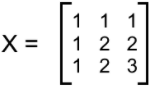1. $\quad$ We start by rewriting the matrix into a notation which is similar to the augmented matrix:
a. $\quad$ We keep the whole matrix $X$ as it is but then instead of a rectangular bracket on the right side, we'll put a vertical line.
b. $\quad$ On the right hand side we put the 3x3 identity matrix.
c. $\quad$ Close the right side of the rectangular bracket.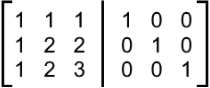2. $\quad$ Having this matrix organization, we can now perform row operations in the matrix in order to find the 3x3 inverse matrix.
a. $\quad$The purpose of this matrix notation is to exchange the identity matrix to the left hand side of the vertical line.
b. $\quad$ In simple words, we are to perform matrix row operations until the left hand side of the matrix looks like the identity matrix.
c. $\quad$ Whatever ends up being the result for each element on the matrix on the right hand side of the vertical line is what will conform the matrix inverse.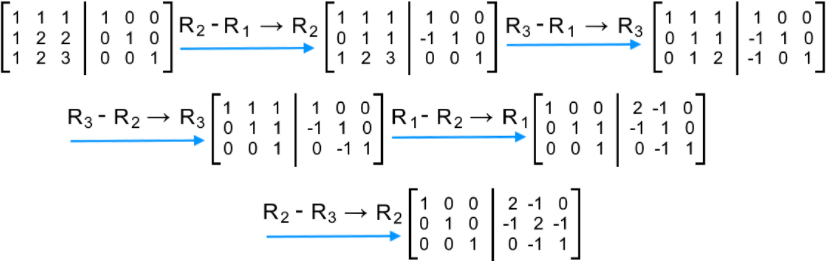3. $\quad$ After the row operations are down, we can transcribe the result on the right hand side of the vertical as the inverse of the original matrix by itself: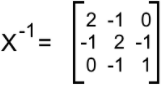And so, in equation 13 we can see the final inverse matrix 3x3.
Let us now work through some example problems so you get practice on the technique.

#### Inverse matrix 3x3 practice problems

For the next example problems, find the matrix inverse 3x3 for each of the given square matrices.

#### Example 1

Given matrix Y as shown below, find its 3x3 matrix inverse.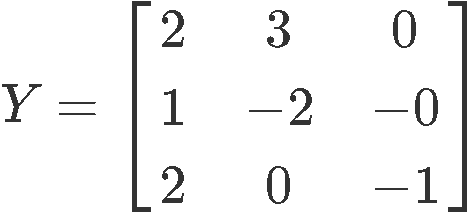We start finding the inverse of a 3x3 matrix such as Y, by rewriting Y together with the third order identity matrix separated by a vertical line, just as studied in the past sections of this lesson. Then, we perform row operations on this matrix until the identity is passed to the left hand side of the vertical line, and the inverse is what is left on the right hand side.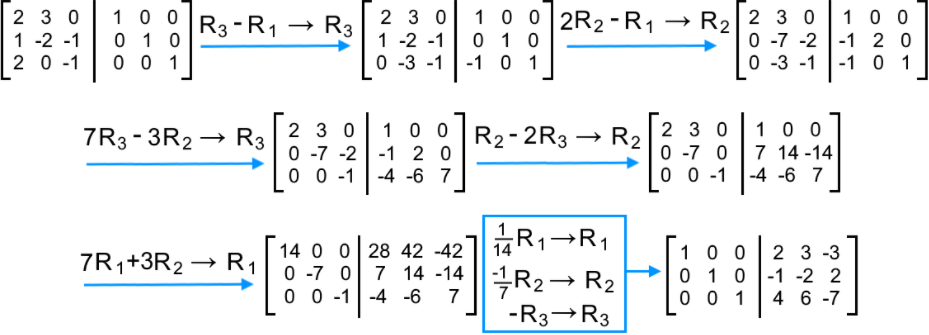Notice the last three row operations were performed at the same time for practicality purposes. And so, the inverse matrix of $Y$ is: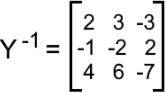#### Example 2

Find the inverse of a 3x3 matrix $R$ as defined below: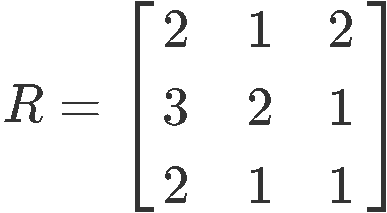To obtain the inverse of 3x3 matrix $R$ we apply the same method as in example 1. Thus, let us start by rewriting R along with the identity matrix to start the inversion and solve through matrix row operations as follows: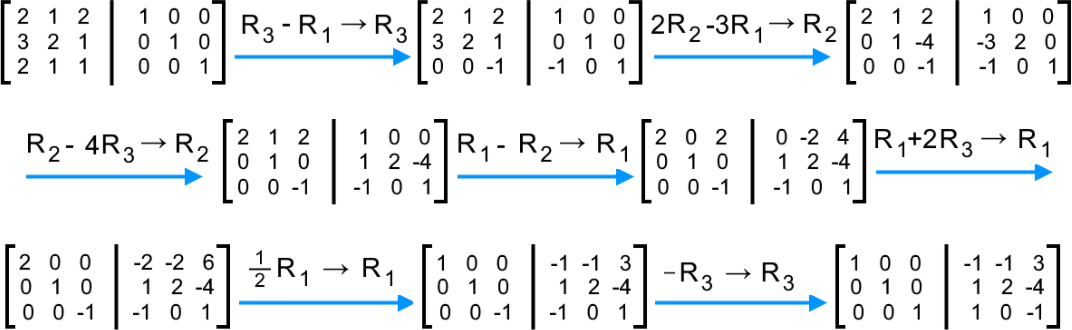And so, the final inverse of matrix $R$ is: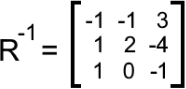#### Example 3

Defining the matrix $A$ as follows: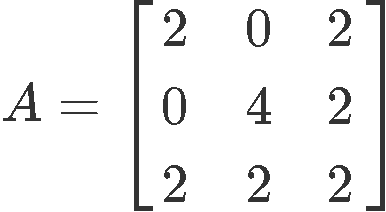Find inverse matrix 3x3 that comes from $A$: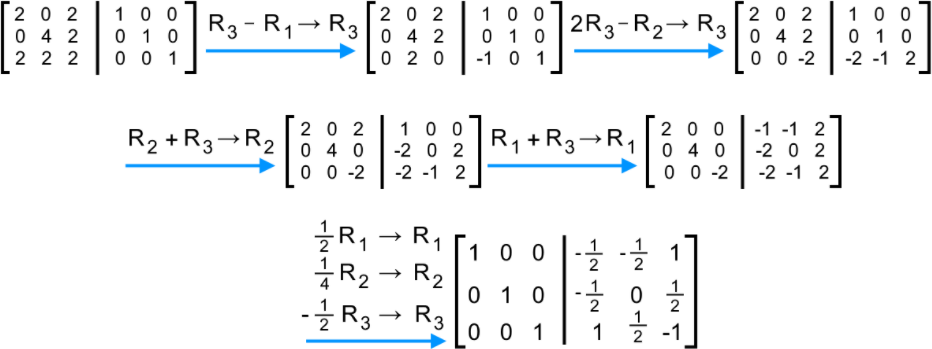And so, after gaussian reduction through row operations we have that the inverse of matrix $A$ is: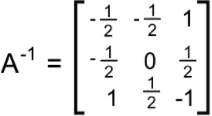#### Example 4

Providing matrix $U$ as defined below: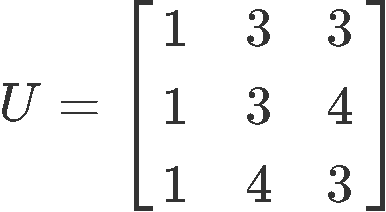Find inverse of 3x3 matrix $U$ by using row operations.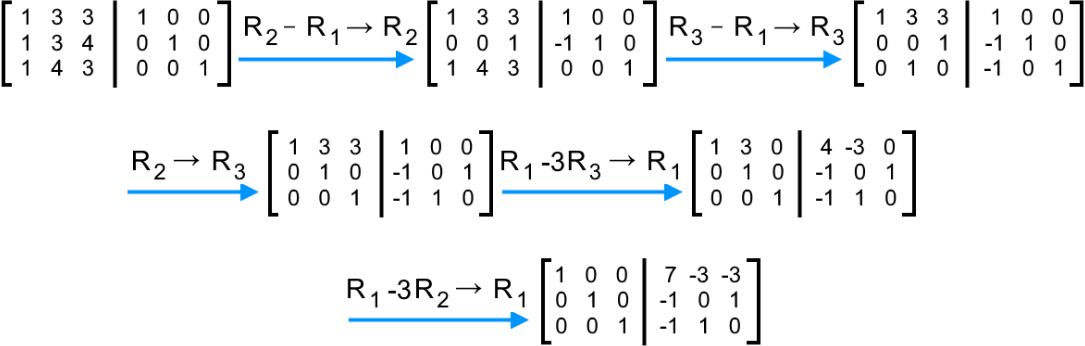And our final result is: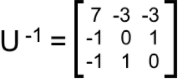#### Example 5

Find inverse of matrix $V$ which is defined as: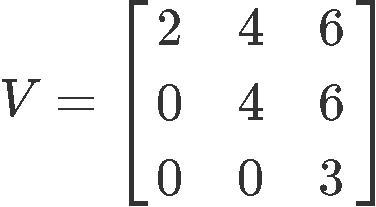Row reducing to obtain the inverse of matrix $V$: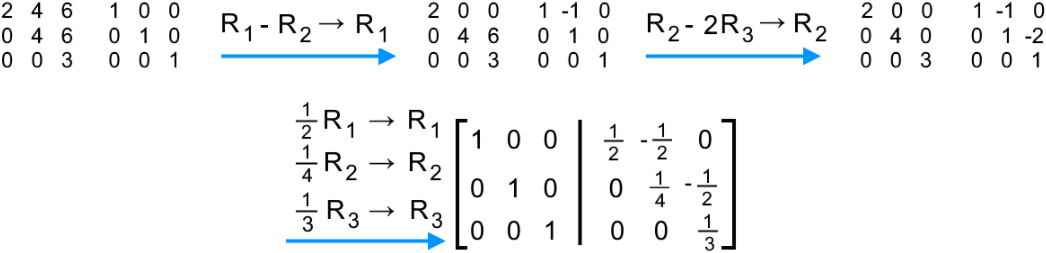We have that the inverse matrix of $V$ is: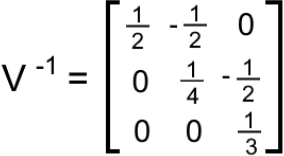***

And so, we hope you have enjoyed this lesson. If you would like to practice the formal method to obtain the inverse of a 3x3 matrix, we recommend you to visit the next link about the inverse of a matrix using minors, cofactors and adjugate. The same can be explained in the next page about the inverse of a 3x3 matrix. Both resources complement our lesson for today, and we recommend you to solve the exercises found here with both methods to complete your studies.

This is it for our lesson today, see you in the next one!

### The inverse of 3 x 3 matrices with matrix row operations

In this section, you will learn how to find the inverse of a 3 x 3 matrix. This method requires the use of matrix row operation. The idea is to draw a vertical line in the middle, write the matrix on the left side of the line, and write the 3 x 3 identity matrix on the right side of the line. Then the goal is to do a bunch of matrix row operation so that the identity matrix appears on the left side of the line. Whatever appears on the right side will be the inverse. Note that this method works for 2 x 2 matrices as well, except the right side of the line is a 2 x 2 identity matrix.

#### Lessons

This is a lot different from finding inverses of 2 x 2 matrices from the previous section, so start with a fresh mind when finding the inverse of 3 x 3 matrices. This method also works for any square matrix!
• Introduction
The Inverse of 3 x 3 Matrix using matrix row operations

• 1.
Finding the Inverse
You are given that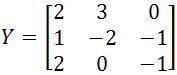. Find the inverse of this matrix.

• 2.
You are given that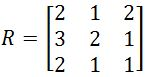. Find the inverse of this matrix.

• 3.
You are given that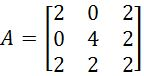. Find the inverse of this matrix.

• 4.
You are given that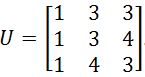. Find the inverse of this matrix.

• 5.
You are given that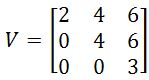. Find the inverse of this matrix.